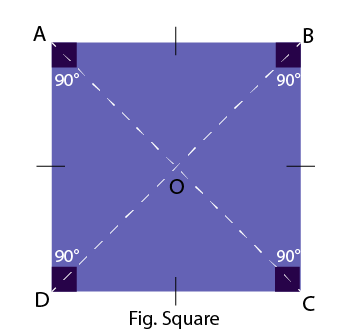To make a square by paper folding and cutting

## Objective

To make a square by paper folding.

### Square

A square is a quadrilateral in which:

• The opposite sides are parallel.
• All four sides are equal.
• All angles measure 90°.### Properties of a Square

• A square is a quadrilateral with four sides and four vertices.
• All four sides of the square are equal.
• The opposite sides of a square are parallel to each other.
• The interior angle of a square at each vertex is 90°.
• The sum of all interior angles is 360°.
• The diagonals of a square bisect each other at 90°.
• The diagonals are equal in length.
• The length of the diagonals in a square is greater than its sides.
• Each diagonal divides a square into two congruent triangles.